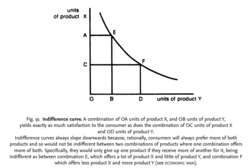# indifference curve

(redirected from Production indifference curve)

## Indifference curve

The expression in a graph of a utility function, where the horizontal axis measures risk and the vertical axis measures expected return. The curve connects all portfolios with the same utility.

## Indifference Curve

A curve on a graph where the x-axis represents a quantity of one good and the y-axis represents a quantity of a second good where the curve represents the universe of quantities with the same utility for a rational investor. The indifference curve is convex, or roughly U-shaped.